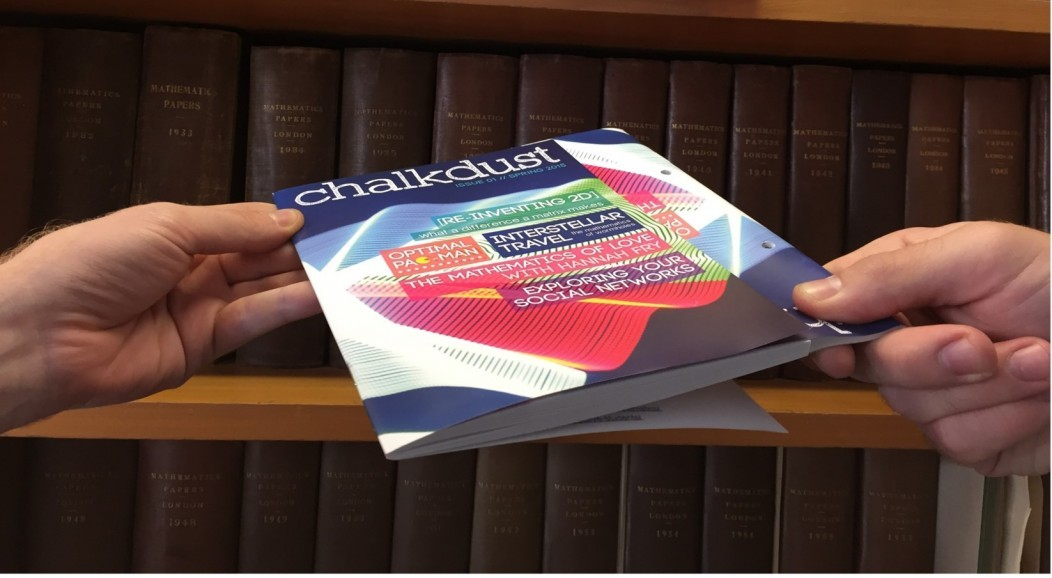# Why is it so hard to pull two phone books apart?

Take two phone books and interleave all their pages one by one. Now try and pull them apart by their spines. Impossible, right?Take two phone books and interleave all their pages one by one. Now try and pull them apart by their spines. Impossible, right? You may have seen this trick before: it’s used as a classic example of the extreme power of friction. There’s some great videos online of two trucks each tethered to one of the books, trying and failing to pull them apart. You can even lift a car with a crane by using a pair of phone books instead of a strap.

The mathematics behind this turns out to be deceivingly complex, and it was only a couple of months ago that academics really worked out what was going on. In August a group of seven physicists, based in France and Canada, posted a paper on the online repository arXiv where they examined this phenomenon in detail, and they found some very interesting results.After reading the paper, I challenged some of my friends at Chalkdust HQ to try to explain roughly what is going on mathematically. Why and how is the friction increasing as you increase the number of pages? Like me, they predicted that friction must be proportional to the contact surface area between the pages: so increasing the number of pages should give an increase in force proportional to the extra surface area of the additional pages.

We couldn’t have been more wrong.

Shamefully we had all forgotten our A level mechanics: it has actually been known since Leonardo da Vinci that friction is not related to the contact area between bodies. Rather it is proportional to the load applied during the sliding between two surfaces. Often this load is due to gravity, in which case the friction will be proportional to the weight of the object lying on the surface. You may remember a picture like this from Mechanics at school where an object is being pulled over a rough surface:The frictional force $F$ is normal to the reaction force $R$, and the relation
\begin{equation*}
F=\mu R
\end{equation*}
holds, where $\mu$ is the coefficient of friction, which changes depending on what the surface is made of. (This always reminds me of a terrible joke: “Why did the cat fall off the roof? Because it didn’t have a large enough $\mu$…”)

So if friction is independent of contact area, where is all this force coming from in the case of the phone books? If you perform the experiment horizontally or vertically the effect is pretty much exactly the same: this means that gravity can only have a tiny effect on the load force. The supreme strength of the system is actually due to a tiny force on the boundary of the phone books, which gets amplified as soon as you try to pull them apart. The force on the boundary is actually due to the elasticity of the pages, which have a slight preference to remain flat. Using a theoretical model, the total traction force $\mathcal{T}$ (i.e. the maximum force you can apply before the pages start slipping apart) was found to vary as
\begin{equation*}
\mathcal{T} = \sqrt{\frac{\pi}{4\alpha}} \exp (\alpha)  \mathrm{erf} (\sqrt{\alpha}).
\end{equation*}
Here $\alpha$ is a dimensionless parameter, which is proportional to the square of the number of pages. The $\textrm{erf}$ function is known as the error function, it frequently appears in statistics and is related to the deviation of a measurement from a normal distribution. In the paper they then tested this formula against experimental results, and it was found to be incredibly accurate. What is remarkable about the formula is that the force varies more than exponentially with page number. There is a particularly striking example mentioned in the paper: increasing the number of pages roughly tenfold (going from 12 sheets to 100) induces an increase in the traction force of  four orders of magnitude.

The crucial ingredient in deriving this result was assuming that the force on the boundary of the books was slightly non-zero, due to the small elasticity of the paper. If not then the solution for the total traction force would also be zero. This emphasises an important point for the applied mathematician: care must always be taken when solving physical problems to choose the boundary conditions appropriately!

There is one sad consequence of this exponential growth. If you try this with a modern day phone book the effect is rather less satisfying than it used to be: the size of phone books seems to decay exponentially by the year. (Damn internet. Although I once spent a summer delivering the Yellow Pages, so I was rather relieved by their smaller size…)

If you want to know more about the details of this phenomenon I encourage you to look at the very readable arXiv paper. Otherwise, I will leave you to enjoy this video of us dangling a textbook from two intertwined copies of Chalkdust Issue 1:Matt is a PhD student at UCL, working in the fields of general relativity and cosmology.
ucl.ac.uk/~ucahawr    + More articles by Matthew